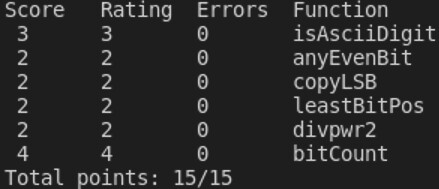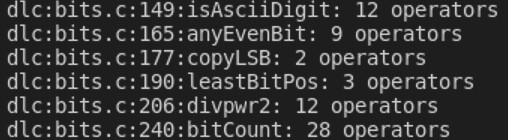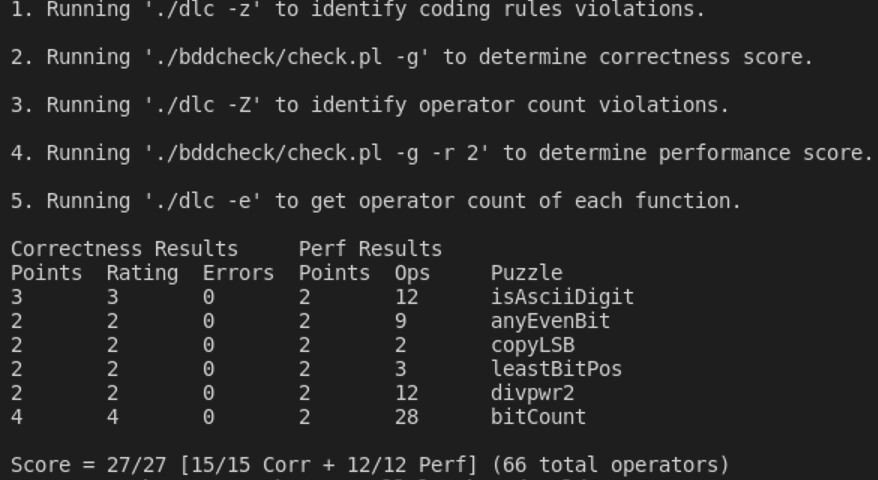Data Lab: Manipulating Bits #1 - 对于实验一（Data Lab）的一些想法（上）。

# Puzzle 1 - isAsciiDigit

isAsciiDigit - return 1 if 0x30 <= x <= 0x39 (ASCII codes for characters ‘0’ to ‘9’)
Examples: isAsciiDigit(0x35) = 1
isAsciiDigit(0x3a) = 0
isAsciiDigit(0x05) = 0
Legal ops: ! ~ & ^ | + << >>
Max ops: 15
Rating: 3

# Puzzle 2 - anyEvenBit

anyEvenBit - return 1 if any even-numbered bit in word set to 1
Examples: anyEvenBit(0xA) = 0, anyEvenBit(0xE) = 1
Legal ops: ! ~ & ^ | + << >>
Max ops: 12
Rating: 2

# Puzzle 3 - copyLSB

copyLSB - set all bits of result to least significant bit of x
Examples: copyLSB(5) = 0xFFFFFFFF, copyLSB(6) = 0x00000000
Legal ops: ! ~ & ^ | + << >>
Max ops: 5
Rating: 2

# Puzzle 4 - leastBitPos

leastBitPos - return a mask that marks the position of the least significant 1 bit. If x == 0, return 0
Example: leastBitPos(0x60) = 0x20
Legal ops: ! ~ & ^ | + << >>
Max ops: 6
Rating: 2

# Puzzle 5 - divpwr2

divpwr2 - Compute x/(2^n), for 0 <= n <= 30
Round toward zero
Examples: divpwr2(15,1) = 7, divpwr2(-33,4) = -2
Legal ops: ! ~ & ^ | + << >>
Max ops: 15
Rating: 2

• x的低n位全部为0时，~x运算后的低n位全部变为1，再加1即“等价于”（对较高位来说）在第n位加1（整体加上1 << n），故~x + 1n位至第31位（认为最低位为第0位，下同）与~x + (1 << n))的相同。则~((~x + 1) >> n) + 1 = ~((~x + (1 << n)) >> n) + 1 = ~(~x >> n + 1) + 1，此即~x >> n + 1的相反数。而~(~x >> n) + 1~x >> n的相反数，即满足~(~x >> n) + 1 + ~x >> n = 0，故所求~x >> n + 1的相反数为~(~x >> n)，即x >> n
• x的低n位不全为0时，即至少某一位为1，~x运算后该位变成0，其他位为1，再加1后的进位一定进到了最低的0上，而不会影响到较高位。故~x + 1n位至第31位~x的相同。则~((~x + 1) >> n) + 1 = ~(~x >> n) + 1 = (x >> n) + 1

# Puzzle 6 - bitCount

bitCount - returns count of number of 1’s in word
Examples: bitCount(5) = 2, bitCount(7) = 3
Legal ops: ! ~ & ^ | + << >>
Max ops: 40
Rating: 4

# Puzzle …WeChat PayAlipay